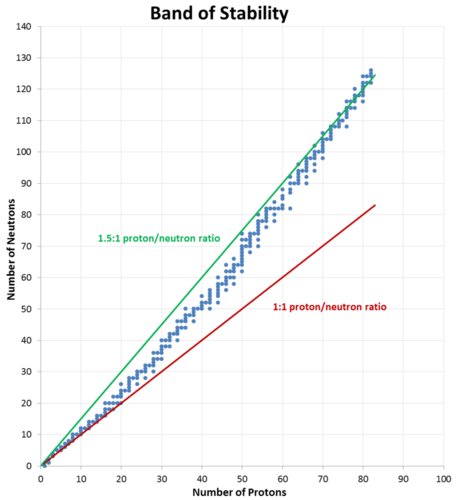11.3: Stable and Unstable Isotopes

Learning Outcomes

• Recognize that radioactivity is a signature of unstable nuclide - radioisotopes.
• Explain how the stability of isotopes depends on the composition of its nucleus.
• Use the "belt of stability" to identify stable isotopes.

The discovery of radioactivity and its effects on the nuclei of elements disproved Dalton's assumption that atoms are indivisible. A nuclide is a term for an atom with a specific number of protons and neutrons in its nucleus. As we will see, when nuclides of one type emit radiation, they are changed into different nuclides. Radioactive decay is spontaneous and does not required an input of energy to occur. The stability of a particular nuclide depends on the composition of its nucleus, including the number of protons, the number of neutrons, and the proton-to-neutron ratio.

In nuclear reactions, it is the nucleus of the atom that gains stability by undergoing a change of some kind. Some elements have no stable isotopes, which means that any atom of that element is radioactive. For some other elements, only certain isotopes are radioactive. A radioisotope is an isotope of an element that is unstable and undergoes radioactive decay. The energies that are released in nuclear reactions are many orders of magnitude greater than the energies involved in chemical reactions. Unlike chemical reactions, nuclear reactions are not noticeably affected by changes in environmental conditions, such as temperature or pressure.

Carbon-12, with six protons and six neutrons, is a stable nucleus, meaning that it does not spontaneously emit radioactivity. Carbon-14, with six protons and eight neutrons, is unstable and naturally radioactive. Among atoms with lower atomic numbers, the ideal ratio of neutrons to protons is approximately 1:1. As the atomic number increases, the stable neutron-proton ratio gradually increases to about 1.5:1 for the heaviest known elements. For example, lead-206 is a stable nucleus that contains 124 neutrons and 82 protons, a ratio of 1.51 to 1.

This observation is shown in Figure $$\PageIndex{1}$$. The band of stability is the range of stable nuclei on a graph that plots the number of neutrons in a nuclide against the number of protons. Known stable nuclides are shown with individual blue dots, while the 1:1 and 1.5:1 ratios are shown with a solid red line and a green line, respectively.Figure $$\PageIndex{1}$$: A graph of the number of neutrons in a nucleus as a function of the number of protons. Each known stable nucleus is represented by a blue dot. The ideal neutron to proton ratio changes from 1:1 for light nuclei to 1.5:1 for the heaviest nuclei.

It should be noted that just because a nucleus is "unstable" (able to undergo spontaneous radioactive decay) does not mean that it will rapidly decompose. For example, uranium-238 is unstable because it spontaneously decays over time, but if a sample of uranium-238 is allowed to sit for 1000 years, only $$0.0000155\%$$ of the sample will have decayed. However, other unstable nuclei, such as berkelium-243, will be almost completely gone (>$$99.9999\%$$ decayed) in less than a day.